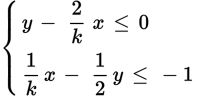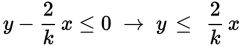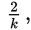# SAT Math Multiple Choice Question 252: Answer and Explanation

### Test Information

Question: 252

12.If the system of inequalities shown has no solution, what is the value of k?

• A. 1
• B. 2
• C. There is no value of k that results in no solution.
• D. There are infinitely many values of k that result in no solution.

Explanation:

D

Difficulty: Hard

Category: Heart of Algebra / Inequalities

Strategic Advice: Some questions cannot be answered using brute force, but rather by understanding how equations and graphs are related. The only way a system of inequalities can have no solution is if the graph consists of two parallel lines with shading in opposite directions so that there is no overlap.

Getting to the Answer: Start by writing each equation in slope-intercept form to help you envision what the graphs will look like. You'll need to multiply the second equation by -2, so don't forget to flip the inequality symbol.Now, think about the graphs. The first equation has a slope ofa y-intercept of 0, and is shaded below the line. The second equation also has a slope ofbut it has a y-intercept of 2 and is shaded above the line. This means that no matter what value of k is used (other than 0), the two lines are parallel and shaded in opposite directions, and thus there are infinitely many values of k that produce a system with no solution.